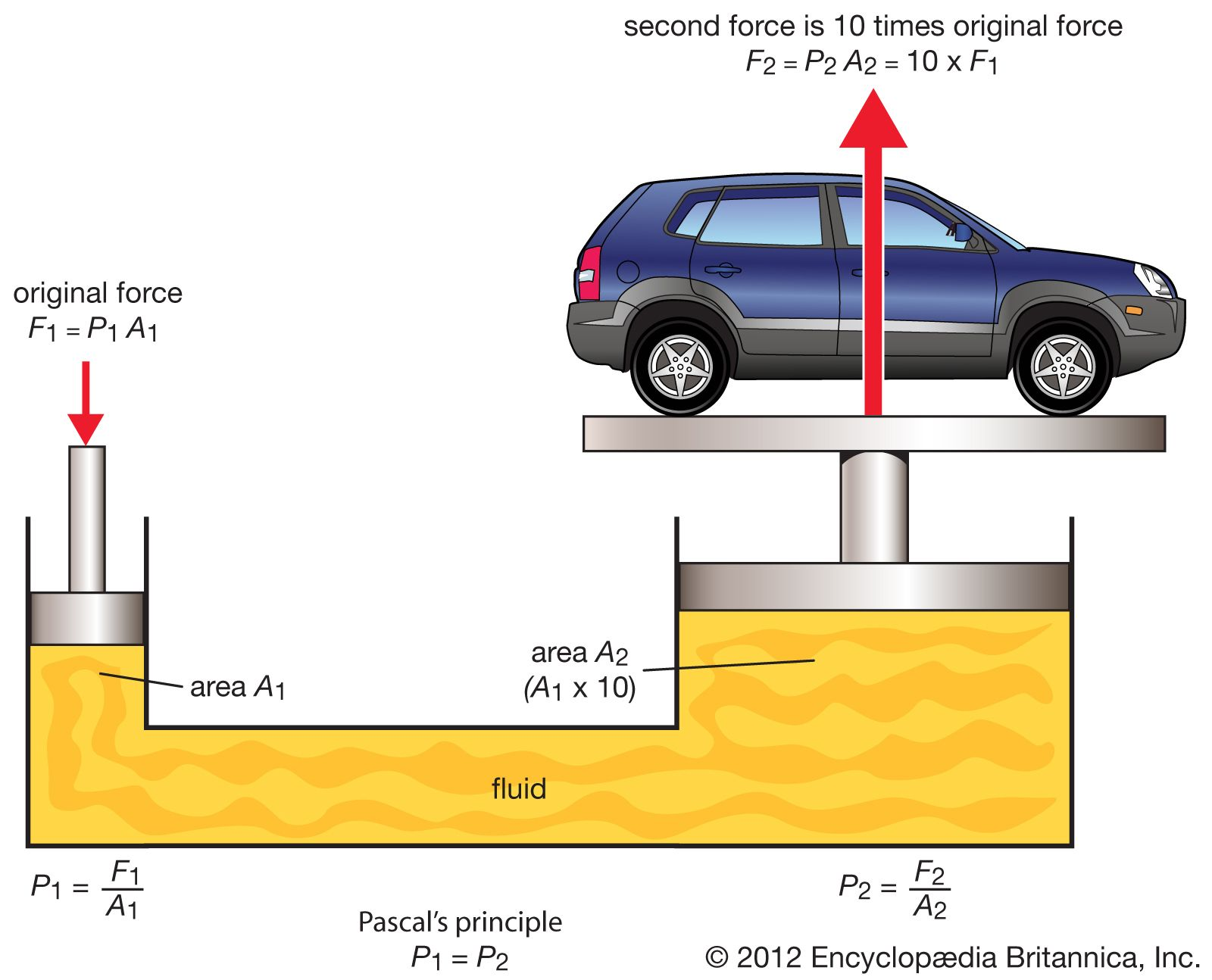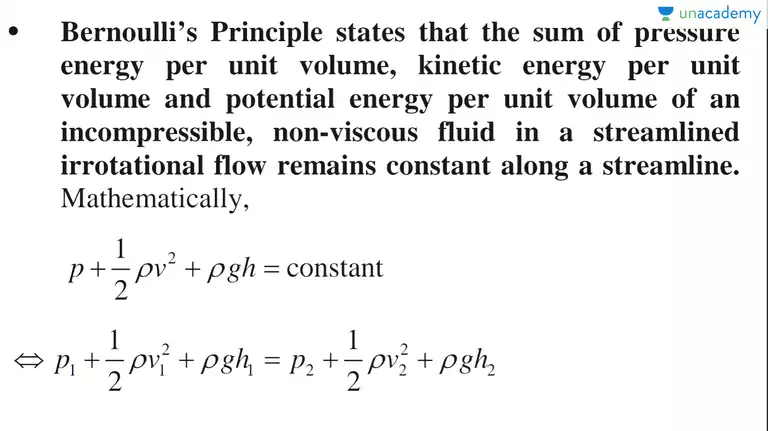# CBSE Class 11 Physics Chapter 10 Revision Notes

Chapter 10: Mechanical Properties of Fluids Revision Notes

• Fluids, like liquids and gases, are substances that can flow. It doesn’t have a defined shape.
• At rest, when an object is submerged in a liquid, the fluid normally exerts a force on its surface. The liquid thrust is what it’s called.
• Pressure: The thrust felt per unit area of a liquid’s surface at rest is known as pressure.
• The force acting on a liquid’s surface is perpendicular everywhere when it is in equilibrium. At the same horizontal level, the pressure is the same.
• The pressure in a liquid depends on the depth (h) below the surface, the density of the liquid, and gravity’s acceleration.

The Law of Pascal:

• The pressure applied to an enclosed liquid is spread to every portion of the liquid and the walls of the containing vessel, according to Pascal’s Law.
• Hydraulic system works on Pascal’s law.
• At all cross sections, the ratio of force exerted to area will be the same.Source

## The Principle of Archimedes

• When a body is submerged partially or completely in a liquid, it loses weight. The weight of the liquid displaced by the immersed part of the body equals the weight of the body in the liquid.
• Buoyancy is the upward force exerted by the liquid displaced when a body is submerged. The body’s weight appears to be decreasing as a result of this.
• A liquid’s flow is said to be steady or stream line if each particle passing through a given point follows the same path and travels at the same speed as the particle before it.
• Laminar flow occurs when a liquid flows over a horizontal surface in layers with different velocities.
• Turbulent flow is defined as a fluid flow in which the velocity of all particles crossing a given point is not the same and the fluid motion becomes irregular or disordered.

Continuity Equation

If there is no fluid source or sink along the length of a pipe, the mass of the fluid crossing any section of the pipe per unit time remains constant, according to the equation of continuity. e.g., a1 v1ρ1 = a2v2 ρ2

A Liquid’s Energy

There are three types of energy in a liquid: kinetic energy, potential energy, and pressure.

• Kinetic energy is the energy possessed by a liquid as a result of its motion, i.e. 1/2mv2.

• P.E. = mgh gives the potential energy of a liquid of mass m at a height h.

• Pressure energy is the energy possessed by a liquid as a result of its pressure. dV = PdV is the pressure energy of a liquid in volume.

Bernoulli’s TheoremSource

The Stokes Law

According to Stokes’ law, the backward dragging force acting on a small spherical body of radius r moving with a velocity v through a viscous medium with coefficient of viscosity is

F = 6πήr

Poiseuille’s Equation

If a pressure difference (P) is maintained across the two ends of a capillary tube with length ‘l’ and radius ‘r,’ the volume of liquid coming out of the tube per second is

(i) directly proportional to the pressure difference, according to Poiseuille (P).

(ii) directly proportional to the fourth power of the capillary tube’s radius (r).

(iii) inversely proportional to the liquid’s coefficient of viscosity (ή).

(iv) inversely proportional to the capillary tube’s length I

• Surface Tension: It is a property of liquids in which the free surface of a liquid at rest tends to have the smallest area and thus behaves like a stretched elastic membrane.
• The force of surface tension is defined as the force acting per unit length of a line drawn on the liquid surface and parallel to it.
]]>